Courses

# RD Sharma Solutions -Ex-9.1, Triangle And Its Angles, Class 9, Maths Class 9 Notes | EduRev

## Class 9: RD Sharma Solutions -Ex-9.1, Triangle And Its Angles, Class 9, Maths Class 9 Notes | EduRev

The document RD Sharma Solutions -Ex-9.1, Triangle And Its Angles, Class 9, Maths Class 9 Notes | EduRev is a part of the Class 9 Course RD Sharma Solutions for Class 9 Mathematics.
All you need of Class 9 at this link: Class 9

Q1) InaΔABC,if∠A = 550,∠B = 400,Find∠C.

Solution:

Given Data:

∠B = 550,∠B = 400,then∠C = ?

We know that

InaΔABC sum of all angles of a triangle is 1800

i.e., ∠A+∠B+∠C = 1800

⇒ 550+400+∠C = 1800

⇒ 950+∠C = 1800

⇒ ∠C = 1800−950

⇒ ∠C = 850

Q2) If the angles of a triangle are in the ratio 1:2:3, determine three angles.

Solution:

Given that,

Angles of a triangle are in the ratio 1:2:3

Let the angles be x, 2x, 3x

∴ We know that,

Sum of all angles of triangles is 1800

x+2x+3x =  1800

⇒ 6x =  1800

⇒ x =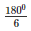⇒ x = 300

Since x = 300

2x = 2(30)0  =  600

3x = 3(30) =  900

Therefore, angles are 300, 600, 900

Q3) The angles of a triangle are (x−400),(x−200)and(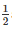x−100). Find the value of x.

Solution:

Given that,

The angles of a triangle are

(x−400),(x−200)and(x−100)

We know that,

Sum of all angles of triangle is 1800

∴(x−400)+(x−200)+(x−100) = 1800

2x+x−70= 1800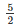x = 1800+700

5x = 2(250)0

x =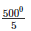∴x = 1000

Q4) The angles of a triangle are arranged in ascending order of magnitude. If the difference between two consecutive angles is 100, find the three angles.

Solution:

Given that,

The difference between two consecutive angles is 100

Let x, x+100, x+200 be the consecutive angles that differ by 100

We know that,

Sum of all angles in a triangle is 1800

x+x+100+x+20 =  1800

3x+300  =  1800

⇒ 3x =  1800– 300

⇒ 3x =  1500

⇒ x =  500

Therefore, the required angles are

x =  500

x+10 =  500 + 100  =  600

x+20 =  50+ 20 =  700

As the difference between two consecutive angles is 100, the three angles are 500,600,700.

Q5) Two angles of a triangle are equal and the third angle is greater than each of those angles by 300. Determine all the angles of the triangle.

Solution:

Given that,

Two angles of a triangle are equal and the third angle is greater than each of those angles by 300.

Let x, x, x+300 be the angles of a triangle

We know that,

Sum of all angles in a triangle is 1800

x + x + x + 300  =  1800

3x + 30 =  1800

3x =  1800−300

3x =  1500

x =  500

Therefore, the three angles are 500,500,800.

Q6) If one angle of a triangle is equal to the sum of the other two, show that the triangle is a right angle triangle.

Solution:

If one angle of a triangle is equal to the sum of the other two angles

⇒ ∠B = ∠A+∠C

In ΔABC,

Sum of all angles of a triangle is 1800

⇒ ∠A+∠B+∠C = 1800

⇒ ∠B+∠B = 1800[∠ B = ∠ A+∠C]

⇒ 2∠B = 1800

⇒ ∠B =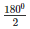⇒ ∠B = 900

Therefore, ABC is a right angled triangle.

Q7) ABC is a triangle in which ∠A = 720, the internal bisectors of angles B and C meet in O. Find the magnitude of ∠BOC.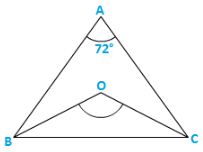Solution:

Given,

ABC is a triangle where ∠A = 720 and the internal bisector of angles B and C meeting O.

In ΔABC,

∠A+∠B+∠C = 1800

⇒ 720+∠B+∠C = 1800

⇒ ∠B+∠C = 1800−720

Dividing both sides by ‘2’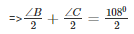⇒ ∠OBC+∠OCB = 540

Now, InΔBOC⇒ ∠OBC+∠OCB+∠BOC = 1800

⇒ 540+∠BOC = 1800

⇒ ∠BOC = 1800−540 = 1260

∴∠BOC = 1260

Q8) The bisectors of base angles of a triangle cannot enclose a right angle in any case.

Solution:

In ΔXYZ,

Sum of all angles of a triangle is 1800

i.e., ∠X+∠Y+∠Z = 1800

Dividing both sides by ‘2’∠X+∠Y+∠Z = 1800∠X+∠OYZ+∠OYZ = 900              [∵ OY, OZ,∠ Y and ∠ Z]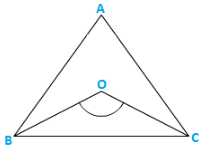⇒ ∠OYZ+∠OZY = 900∠X

Now in ΔYOZ

∴∠YOZ+∠OYZ+∠OZY = 1800

⇒ ∠YOZ+900∠X = 1800

⇒ ∠YOZ = 900∠X

Therefore, the bisectors of a base angle cannot enclosure right angle.

Q9) If the bisectors of the base angles of a triangle enclose an angle of 1350, prove that the triangle is a right angle.

Solution: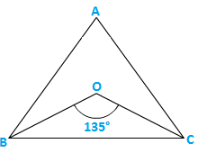Given the bisectors of the base angles of a triangle enclose an angle of 1350

i.e., ∠BOC = 1350

But, We know that

∠BOC = 900+∠A

⇒ 1350 = 900+∠A∠A = 1350−900

⇒ ∠A = 450(2)

⇒ ∠A = 900

Therefore, ΔABC is a right angle triangle that is right angled at A.

Q10) In a ΔABC, ∠ABC = ∠ACB and the bisectors of ∠ABCand∠ACB intersect at O such that ∠BOC = 1200. Show that ∠A = ∠B = ∠C = 600.

Solution: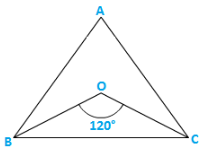Given,

In ΔABC,

∠ABC = ∠ACB

Dividing both sides by ‘2’∠ABC =∠ACB

⇒ ∠OBC = ∠OCB                                              [∴OB,OC bisects ∠B and ∠C]

Now,

∠BOC = 900+∠A

⇒ 1200−90=∠A

⇒ 300∗(2) = ∠A

⇒ ∠A = 600

Now in ΔABC

∠A+∠ABC+∠ACB = 1800(Sumofallanglesofatriangle)

⇒ 600+2∠ABC = 1800                        [∴∠ABC = ∠ACB]

⇒ 2∠ABC = 1800−600

⇒ ∠ABC =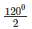= 600

⇒ ∠ABC = ∠ACB

∴∠ACB = 600

Hence Proved.

Q11)  Can a triangle have:

(i) Two right angles?

(ii) Two obtuse angles?

(iii) Two acute angles?

(iv) All angles more than 60°?

(v) All angles less than 60°?

(vi) All angles equal to 60″?

Sol:

(i) No,

Two right angles would up to 180°. So the third angle becomes zero. This is not possible, so a triangle cannot have two right angles. [Since sum of angles in a triangle is 1800]

(ii) No,

A triangle can’t have 2 obtuse angles. Obtuse angle means more than 90° So that the sum of the two sides will exceed 180° which is not possible. As the sum of all three angles of a triangle is 180°.

(iii) Yes

A triangle can have 2 acute angles. Acute angle means less the 90″ angle.

(iv) No

Having angles more than 600 make that sum more than 1800. This is not possible.  [Since the sum of all the internal angles of a triangle is 1800]

(v) No

Having all angles less than 600 will make that sum less than 1800 which is not possible.[Therefore, the sum of all the internal angles of a triangle is 1800]

(vi) Yes

A triangle can have three angles equal to 600 . Then the sum of three angles equal to the 1800.  Such triangles are called as equilateral triangle. [Since, the sum of all the internal angles of a triangle is1800]

Q12) If each angle of a triangle is less than the sum of the other two, show that the triangle is acute angled.

Solution

Given each angle of a triangle less than the sum of the other two

∴∠X+∠Y+∠Z

⇒ ∠X+∠X<∠X+∠Y+∠Z

⇒ 2∠X<1800                           [Sumofalltheanglesofatriangle]

⇒ ∠X<900

Similarly ∠Y<900and∠Z<900

Hence, the triangles are acute angled.

The document RD Sharma Solutions -Ex-9.1, Triangle And Its Angles, Class 9, Maths Class 9 Notes | EduRev is a part of the Class 9 Course RD Sharma Solutions for Class 9 Mathematics.
All you need of Class 9 at this link: Class 9Use Code STAYHOME200 and get INR 200 additional OFF Use Coupon Code
All Tests, Videos & Notes of Class 9: Class 9

### Up next### Top Courses for Class 9## RD Sharma Solutions for Class 9 Mathematics

91 docs

### Up next### Top Courses for Class 9Track your progress, build streaks, highlight & save important lessons and more!

,

,

,

,

,

,

,

,

,

,

,

,

,

,

,

,

,

,

,

,

,

,

,

,

,

,

,

,

,

,

;